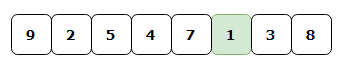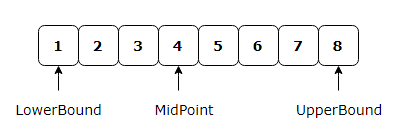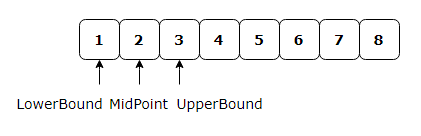# Searching Algorithms In C#

## Introduction

In this article, I am going to discuss two of the most commonly-used searching algorithms in the programming world

1. Linear Search
2. Binary Search

I will be explaining the algorithms with the help of an example and will provide a C# code to execute that.

## Linear Search

This algorithm will perform a sequential search of item in the given array. Every element is checked from start to end and if a match is found, the index of matched element will be returned; otherwise, -1 will be returned.

### Pseudo Code for Linear Search

```procedure LinearSearch(array, value)

foreach item in array
if item == value
return the item's index
end if
end foreach
return -1

end procedure ```

Let us understand this with the help of an example.

Consider the array below.If we want to determine the position of number 1 in this array, we have to traverse every element from start to end; i.e from index=0 to index = 7 and compare it with 1. We will return the position of element which matches with 1, which is 6 (index+1). Hence, the element 1 is found at position 6 in input array.

### Time Complexity

Since all the array elements are compared only once with the input element, hence the time complexity of the linear search is O(N).

## Binary Search

Binary search is an efficient and commonly used searching algorithm.This algorithm works only on sorted sets of elements. So if the given array is not sorted then we need to sort it before applying Binary search.

This algorithm searches a sorted array by repeatedly dividing the search interval in half. Begin with an interval covering the whole array. If the value of the search key is less than the item in the middle of the interval, narrow the interval to the lower half. Otherwise narrow it to the upper half. Repeatedly check until the value is found or the interval is empty.if found return the index of matched element , else return -1.

### Pseudo Code for Binary Search

```Procedure BinarySearch(array,value)

Set lowerBound = 1
Set upperBound = size of array

while (lowerBound <= upperBound)
set midPoint = (lowerBound + upperBound ) / 2
if array[midPoint] > value
set upperBound = midPoint - 1
else if array[midPoint] < value
set lowerBound = midPoint + 1
else return midPoint
end while

return -1;

end procedure```
Let us understand this with the help of an example.

Referring to the image below; if we need to find the position of 2 in the given array using binary search ,The lower bound is 0 and the upper bound is 7. The median of the lower and upper bounds is (lower_bound + upper_bound) / 2 = 3. Here array = 4. The value mid element (4) is greater than the value to be found(2). Therefore, we do not need to conduct a search on any element beyond 4 as the elements beyond it will obviously be greater than 2.

Therefore, we drop the upper bound of the array to the position of element just before the mid element. Now, we follow the same procedure on the same array with the following values,Now, the lower bound is 0 and the upper bound is 2. The median of the lower and upper bounds is (lower_bound + upper_bound) / 2 = 1. Here array = 2.Hence the value of mid element matches the value to be searched.Therefore we return the position of 2 as 2.

### Time Complexity

The array to be searched is reduced by half in every iteration. Hence time complexity of the Binary search is O(LogN).

## Linear Search vs Binary Search

Linear Search is sequential search which scans one item at a time.The time taken to search a given element will increase if the number of elements in the array increases.

For Binary Search the input array needs to be in sorted order. It also accesses the data randomly as it always compares to the middle element of array and hence discarding the half of array in every iteration.

Linear search performs equality comparisons whereas Binary search performs ordering comparisons